# The only thing you need to know to fold any odd division grid

What if I told you that you don’t need to look up how to fold thirds, fifths, or even 17ths ever again? Like you, I used to spend a lot of time looking up how to fold thirds every time I wanted to fold a 48-fold grid. No more.

I learned this method from Elseh (@zamanulli on IG) back in May 2020 and I've been using it for my odd grids ever since.

### Tell me more

So, let’s get down to it: what’s this awesome piece of knowledge that will change your folding life?

Basic unit of knowledge: The sum of the grid spacings between any two pairs of points on parallel lines gives you the number of divisions you can make between the parallel lines with references from only those four points.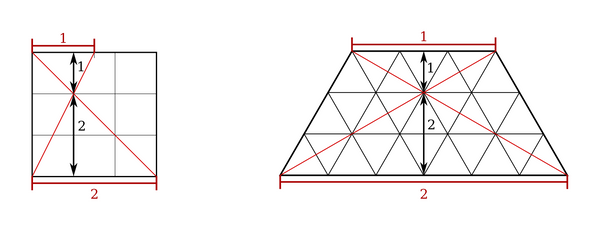More specifically: If you take a top line and a bottom line on your existing grid and count out a certain number of spacings $// $ on the top and a certain (different) number of spacings $// $ on the bottom, then diagonals across the trapezoid formed by the boundaries of your top and bottom spacings will cross where you have $// $ divisions below the intersection and $// $ divisions above it.

Another key point is that if a third side of this trapezoid is on an existing grid line, then the marked intersection will lie on a grid intersection of the odd division and so can be used as a reference in all directions. This is true of both square grids and triangle grids.

### Ok, but how do I use this?

1. Pick your points so that the grid spacings on the bottom and the grid spacings on the top add up to your target number. For these examples, I’ll take 2 spacings on the bottom and 1 spacing on the top to get a division by 2+1 = 3.

• Caveat: these spacings can’t have common divisors. For example, 6 and 3 will just give you thirds, not ninths, because both 6 and 3 are divisible by 3. For ninths, you’d need to pick 1 and 8, 2 and 7, 4 and 5, or do thirds twice.
• Fold the diagonals between your four points. You don’t need to fold all the way - just pinch near the intersection if you want a cleaner fold.

• Since you’re folding an odd division in the grid, one of the sides of the intersection will have an even number of divisions.

• How do I know this? Only an odd number and an even number can add to make an odd number. Both odd-odd and even-even addition give even numbers.
• Fold the line on the even side to meet the point marked by the intersection of the diagonals. In this case, I fold the bottom line up to the intersection.

• This may mean folding a line to a point, which is a less accurate method than point to point. Keep an eye out for additional references you can use to make sure your new fold is parallel to your existing grid.
• If the side you just folded still has gaps divisible by 2, keep dividing it until it has odd divisions.

• Then take the other side and fold it to the first crease after the marked intersection. This will be an even division since you started with an odd number and you’re adding an odd number.

• Continue until you’ve filled in all the creases.

• If your grid already had several divisions before you performed the odd division, propagate the odd division through the rest of the paper.

• Complete your odd grid division by dividing all the other grid directions in the same odd division.Let’s run through this for a particularly involved example: 7-fold divisions with spacings of 4 and 3.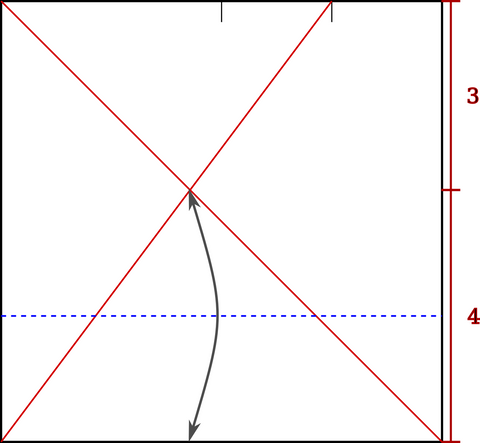I start with two pinches at the top to mark $// $ and $// $ of the way across, and fold my two diagonals. One of my diagonals is the main diagonal of the square, which lets me reuse this intersection point as a reference for the other direction too.

The area below the intersection has 4 divisions, so I fold it in half.The area below the intersection has spacings of 2, so I fold those in half again.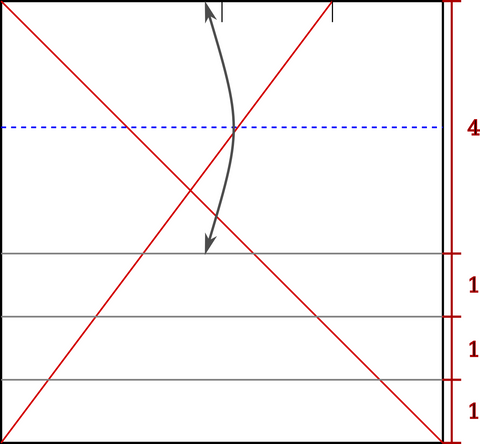Now the distance between the top and the closest crease is even, so I fold that in half.There’s still even gaps in the top, so I fold those in half too. Now the 7ths are done in that direction, and I can repeat the process with the same reference intersection in the other direction!

It’s easier to fold the odd divisions of a grid early in the gridding process, before there’s a ton of lines to keep track of. So, if you’re folding a 48-fold grid, don’t fold four divisions of 2 and then divide by 3 - fold in half once, then divide by 3, then do another three divisions of two.

### Ok, this sounds pretty great - where’s the proof?

We’ll go through this in three stages. Our goal is to prove that the intersection splits our paper into a lower section proportional to $// $ and an upper section proportional to $// $ , which means that the height of the intersection should be proportional to the distance between the lines and to $// $ . We’ll take the lower left corner as $// $ in all three cases.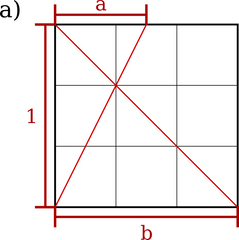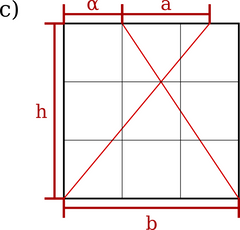In case a), the bottom points have coordinates $// $ and $// $ and the top points have coordinates $// $ and $// $ . this makes the formulas for the two diagonals

which rearrange to give

Setting these equal at the intersection, we get

which is precisely what we wanted.

Let’s also solve for $// $ , which will tell us whether we’ve found a reference for both directions.

Since we’ve established that $// $ is some multiple of the desired division, then $// $ must also be a multiple of the division since $// $ is an integer ( $// $ ) multiple of $// $ .

Moving on to case b), the bottom points are the same and the top points have height $// $ . So, the two diagonals have formulas

which rearrange to give

Setting these equal at the intersection, we get

which is again precisely as expected.

Solving for $// $ , we get exactly the same result as before.

This shows that no matter what value we have for $// $ , the $// $ value of the intersection of the diagonals stays the same.

Our final case, c), represents the most arbitrary possible selection of points, the general case. This is what we use on triangle grids.

The bottom points are again the same, and the top points have been shifted right by $// $ , and have coordinates $// $ and $// $ . So, the two diagonal have the formulas

which rearrange to give

Setting these equal at the intersection, we get

which is again exactly what we expected and proves the general case for the vertical direction.

Let’s take a look at the horizontal direction in the general case. First, let’s solve for $// $ .

Again, we get that $// $ is independent of $// $ , but this time there is a dependence on $// $ . So, when will $// $ land on a new grid intersection and be a useful reference in all directions?

Let’s look first at what makes a grid a grid - consistent spacing in defined directions. Using just two directions that aren’t the same and distances in each direction, I can define the relationship between any two points in terms of those two directions and distances. In linear algebra, we call these directions and distances basis vectors and for a 2D plane each vector will have $// $ and $// $ components, which are written $\begin{bmatrix}x\\y\end{bmatrix}$.

The basis vectors for square grids are $// $ and $// $ when grid spacings equal 1, or in the case of odd divisions $// $ and $// $ .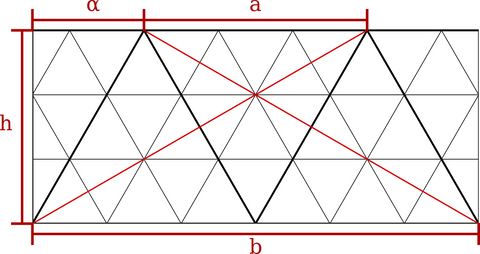For triangle grids I’ll choose the horizontal and upper right diagonal lines as basis vectors, which are $// $ and $// $ when grid spacings equal 1, or in the case of odd divisions $// $ and $// $ .

If the intersection point of the diagonals of the trapezoid lands on a grid intersection, then it must be composed of integer multiples of the basis vectors of the underlying grid.

We already established that the first basis vector for both square grid and triangle grid is $// $ , so we can fill in $// $ and $// $ .

Looking at the triangle grid case, we see that the setup for the basis vector is remarkably similar to our trapezoid - in fact, if the target point of our second basis vector is exactly the top left point of the trapezoid, then the second basis vector can be restated as $// $ , with $// $ as the odd division case.

Using these basis vectors, we can solve for $// $ and $// $ .

Since the first basis vector doesn’t contribute at all to the $// $ value of the intersection, we have

which we can then plug in to find $// $ .

Ok, so we’ve found a basis vector system that works to find our reference intersection. What does that mean for the trapezoids we can choose to define the references?

As long as $// $ is a basis vector of the original grid, the reference intersection will be on an odd grid intersection. In the square grid case, that means that $// $ must equal 0.

Stated more plainly, both of the parallel lines used for the odd division and one other edge of the trapezoid need to already be on grid lines to make the intersection of the diagonals of the trapezoid fall on an odd grid intersection. The final edge of the trapezoid does not need to be on a grid line (and usually won’t be) and we don’t care whether that final edge is on the left or right side of the trapezoid.

If you use this in your own folding, go ahead and share it with me (@gatheringfolds) on Instagram or Facebook! I love to see people folding grids - and the tessellations made with them!

Also, if you have any questions about this post or any other gridding topics that you’d like me to explore next, send your questions and topics to madonna[at]gatheringfolds.com and I’ll see what I can do to help!

• We need a grid because we don’t have a laser/scoring machine to cut the exact crease pattern and also because the exact crease pattern is harder to fold anyways!
The secret upside to starting from a grid is that it’s easier to find new patterns too – you’re not locked into a specific design from the beginning.